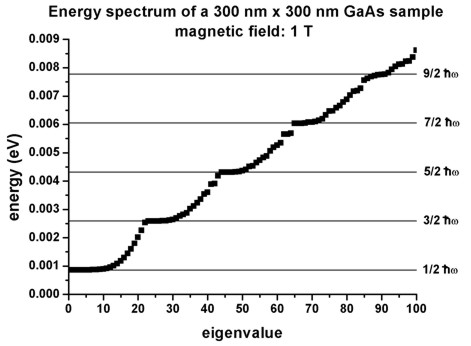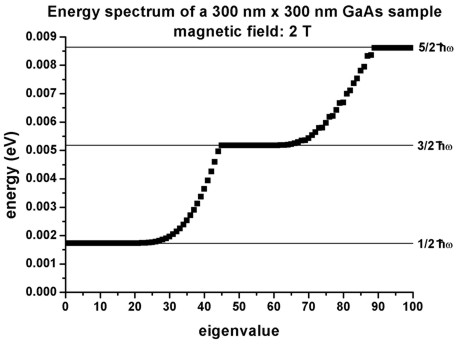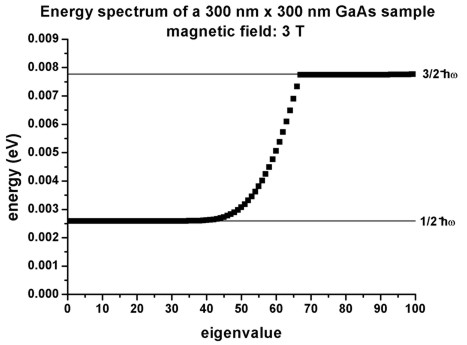nextnano.com  GUI: nextnanomat  Tool: nextnano++  Tool: nextnano³  Tool: nextnano.NEGF  Download | Search | Copyright | News | Publications  * password protected nextnano³ software2D Landau levels

# nextnano3 - Tutorial

## Landau levels of a bulk GaAs sample in a magnetic field

Author: Stefan Birner

If you want to obtain the input files that are used within this tutorial, please contact stefan.birner@nextnano.de.
` -> 2DBulkGaAs_LandauLevels_nn3.in / *_nnp.in - `input file for the nextnano3 and nextnano++ software```  ```

## Landau levels of a bulk GaAs sample in a magnetic field

In this tutorial we study the electron energy levels of a bulk GaAs sample that is subject to a magnetic field.

• The GaAs sample extends in the x and y directions (i.e. this is a two-dimensional simulation) and has the size of 300 nm x 300 nm.
At the domain boundaries we employ Dirichlet boundary conditions to the Schrödinger equation, i.e. infinite barriers.

• The magnetic field is oriented along the z direction, i.e. it it perpendicular to the simulation plane which is oriented in the (x,y) plane).
We calculate the eigenstates for different magnetic field strengths (1 T, 2 T, 3 T), i.e. we make use of the magnetic field sweep.

```  \$magnetic-field    magnetic-field-on                    = yes    magnetic-field-strength              = 0.0     ! ```0 Tesla = 0 Vs/m2```    magnetic-field-direction             = 0 0 1   ! ``` direction```    magnetic-field-sweep-active          = yes     !    magnetic-field-sweep-step-size       = 1.0     ! ```1 Tesla = 1 Vs/m2```    magnetic-field-sweep-number-of-steps = 3       ! ```3 magnetic field sweep steps```    output-magnetic-vector-potential     = yes     ! ```output of A(x,y,z)```   \$end_magnetic-field```

• A useful quantitiy is the magnetic length (or Landau magnetic length) which is defined as:
lB = [hbar / (me* wc)]1/2 = [hbar / (|e| B)]1/2
It is independent of the mass of the particle and depends only on the magnetic field strength:
`- 1 T: `25.6556 nm
`- 2 T: `18.1413 nm
`- 3 T: `14.8123 nm

• The electron effective mass in GaAs is me* = 0.067 m0. Another useful quantity is the cyclotron frequency:
wc = |e| B / me*
Thus for the electrons in GaAs, it holds for the different magnetic field strengths:
`- 1 T: `hbarwc = 1.7279 meV
`- 2 T: `hbarwc = 3.4558 meV
`- 3 T: `hbarwc = 5.1836 meV

• The energy spectra for different magnetic fields (1 T, 2 T, 3 T) look as follows:• The Landau levels are given by En = (n - 1/2) hbarwc where n = 1,2,3,...

• The number of states for each Landau level can be calculated as follows (see P.Y. Yu, M. Cardona, Fundamentals of Semiconductors, p. 536, 3rd ed.):
N = LxLy |e| B / h = 1/(2pi)  LxLy / lB2        (ignoring spin)
where Lx and Ly are the lengths in the x and y directions (300 nm in this example) and lB is the magnetic length.

N(1 T) = 21.76` ==> ~`22 states per Landau level (in the figure above: 22 as it should be)
N(2 T) = 43.52` ==> ~`44 states per Landau level (in the figure above: 44 as it should be)
N(3 T) = 65.29` ==> ~`66 states per Landau level (in the figure above: 66 as it should be)

Note that N is independent of n.
• For the calculations, we used the symmetric gauge A = `-` 1/2 r x B = 1/2 B x r
leading to the following energies (see J.H. Davies, The Physics of Low-Dimensional Semiconductors, p. 222):
En,l = (n + 1/2 l + 1/2 |l| - 1/2) hbarwc
One can see that all states having a negative value of 'l' are degenerate with the states with l=0, i.e. the allowed energies are independent of l if l < 0 (for the same n).
The energies increase if l increases (for l > 0 and for the same n).

• The motion in the z direction is not influenced by the magnetic field and is thus that of a free particle with energies and wave functions given by:
Ez = hbar2 kz2 / (2 m*)
psi(z) = exp (+`-` i kz z)
For that reason, we did not include the z direction into our simulation domain, and thus only simulate in the (x,y) plane (two-dimensional simulation).

• Please help us to improve our tutorial. Send comments to``` support [at] nextnano.com```.